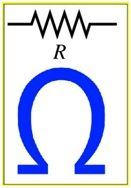# Resistance Formula

## Resistance

Resistance is the measure of opposition applied by any object to the flow of electric current. A resistor is an electronic constituent that is used in the circuit with the purpose of offering that specific amount of resistance.The resistance of any object is computed making use of the formula:

$$\begin{array}{l}R=\frac{V}{I}\end{array}$$

In this case,
v = Voltage across its ends
I = Current flowing through it

If the resistance’s are linked in series, the series resistance is articulated as-

$$\begin{array}{l}R_{s}=R_{1}+R_{2}+R_{3}+…….+R_{n}\end{array}$$

If resistance is connected in parallel, the parallel resistance is given by

$$\begin{array}{l}\frac{1}{R_{p}}=\frac{1}{R_{1}}+\frac{1}{R_{2}}+\frac{1}{R_{3}}+……+\frac{1}{R_{n}}\end{array}$$

Where, R1, R2, R3 …… Rn is the given resistors. Resistance is measured in ohms (Ω).

Resistance Formula is used to compute the resistance across any given number of resistors in the circuit. It can similarly be made use of to compute the resistance in any given object.

### Solved Examples

Below are problems based on the resistance that may be helpful for you.

Example 1: Compute the resistance offered by the body if 2mA of current is flowing having a potential difference of 2V?

Known:

I (Current) = 2mA,
V (Potential difference) = 2 V

The resistance is given by:

$$\begin{array}{l}R=\frac{V}{I}=\frac{2}{2\times 10^{-3}}=1000\Omega\end{array}$$

Example 2: Compute the resistance if 5 Ω and 2 Ω are connected in parallel.

Known:

Resistance

R= 5 Ω,

R2 = 2 Ω

The parallel resistance is articulated as

$$\begin{array}{l}\frac{1}{R_{p}}=\frac{1}{R_{1}}+\frac{1}{R_{2}}\end{array}$$

$$\begin{array}{l}R_{p}=\frac{R_{1}R_{2}}{R_{1}+R_{2}}=\frac{5 \times 2}{5+2}=1.428\Omega\end{array}$$

Stay tuned with BYJU’S for more such interesting articles. Also, register to “BYJU’S – The Learning App” for loads of interactive, engaging Physics-related videos and unlimited academic assist.